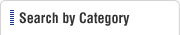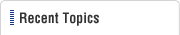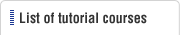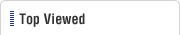HOME  > Press Dies Tutorial  > #126 Press Forming Force (3) Bending Force
Press Dies Tutorial

#126 Press Forming Force (3) Bending Force

Category : Blanking
June 1, 2012

The bending force discussed here is the force of forming a free bend. In bending operations, bottom bending is used frequently at the bottom dead center in order to stabilize the shape. An extremely large force is required in bottom bending depending on the amount of bottoming. The size of that force is considered to be 5 to 10 times the free bending force.

#### (1) Bending force for V-bending (See Fig. 1)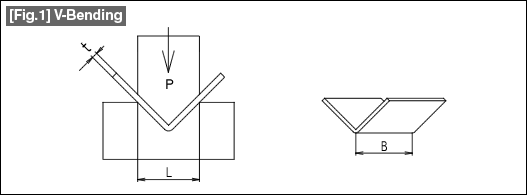The bending force for V-bending is obtained using the following equation.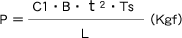P: Bending force (kgf) 　　C1: Coefficient 　　B: Bending line length (mm) 　　t: Plate thickness (mm) 　　Ts: Tensile strength（Kgf/mm2） 　The coefficient (C1) is 1.33 when the die shoulder width (L) is 8 times the material plate thickness (t), 1.5 when the die shoulder width is about 5 times the plate thickness, and about 1.2 when it is about 16 times the plate thickness.

#### (2) Bending force for L-bending (see Fig. 2)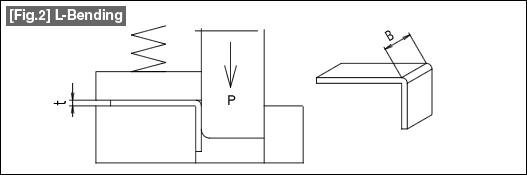The bending force for L-bending is obtained using the following equation.

 P=C/3 * B * t * Ts（Kgf） 　　P: Bending force (kgf) 　　C: Coefficient ... 1.0 to 2.0 　　　(Select a larger coefficient when the punch R and the Die R are small.) 　　B: Bending line length (mm) 　　t: Plate thickness (mm) 　　Ts: Tensile strength（Kgf/mm2）

 This shape is taken as the basis for the bending force for pad-pressed bending. In the case of U-bending such as shown in Fig. 3, since the bending line (B) is present at two locations, the above calculation is made by doubling the vale of the bending line length (B) in the above equation.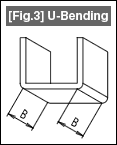In this manner, when bending is done in several locations at the same time, the total length of the bending lines is taken as the value of B.